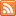# Content Tagged “Exploratory Data Analysis”Exploratory data analysis (or descriptive statistics) is used to summarize the collected data. EDA consists of organizing and summarizing the raw data,
discovering important features and patterns in the data and any striking deviations from those patterns, and then interpreting our findings in the context of the problem.

This can be useful for describing the distribution of a single variable (center, spread, shape, outliers), checking data (for errors or other problems), checking assumptions to more complex statistical analyses, and investigating relationships between variables.## Boxplots

Published: Aug 21st, 2012

The Five Number Summary The Boxplot Side-By-Side (Comparative) Boxplots Let’s Summarize CO-4: Distinguish among different measurement scales, choose the appropriate descriptive and inferential statistical methods based on these distinctions, and interpret […]## Outliers

Published: Aug 21st, 2012

Using the IQR to Detect Outliers The 1.5(IQR) Criterion for Outliers The 3(IQR) Criterion for Outliers Understanding Outliers CO-4: Distinguish among different measurement scales, choose the appropriate descriptive and inferential statistical […]Published: Aug 21st, 2012

Introduction Range Inter-Quartile Range (IQR) Standard Deviation Properties of the Standard Deviation Choosing Numerical Measures Let’s Summarize CO-4: Distinguish among different measurement scales, choose the appropriate descriptive and inferential statistical methods […]## Measures of Center

Published: Aug 21st, 2012

Introduction Mean Median Comparing the Mean and the Median Let’s Summarize CO-4: Distinguish among different measurement scales, choose the appropriate descriptive and inferential statistical methods based on these distinctions, and interpret […]## Histograms & Stemplots

Published: Aug 19th, 2012

Histograms Stemplot (Stem and Leaf Plot) Let’s Summarize CO-4: Distinguish among different measurement scales, choose the appropriate descriptive and inferential statistical methods based on these distinctions, and interpret the results. LO […]

## One Quantitative Variable

Published: Aug 19th, 2012

Distribution of One Quantitative Variable Graphs Numerical Measures CO-4: Distinguish among different measurement scales, choose the appropriate descriptive and inferential statistical methods based on these distinctions, and interpret the results. Video: One […]## One Categorical Variable

Published: Aug 4th, 2012

Distribution of One Categorical Variable Numerical Measures Visual or Graphical Displays Pie Chart Bar Chart Pictograms Let’s Summarize CO-4: Distinguish among different measurement scales, choose the appropriate descriptive and inferential statistical […]## Unit 1: Exploratory Data Analysis

Published: Jul 30th, 2012

The Big Picture Exploratory Data Analysis Important Features of Exploratory Data Analysis Examining Distributions CO-1: Describe the roles biostatistics serves in the discipline of public health. CO-6: Apply basic concepts of […]## The Big Picture

Published: Jul 30th, 2012

CO-1: Describe the roles biostatistics serves in the discipline of public health. Throughout the course, we will add to our understanding of the definitions, concepts, and processes which are introduced here. […]## Unit 3A: Probability

Published: Jul 28th, 2012

Review of the Big Picture Probability and Inference CO-1: Describe the roles biostatistics serves in the discipline of public health. CO-6: Apply basic concepts of probability, random variation, and commonly used statistical […]